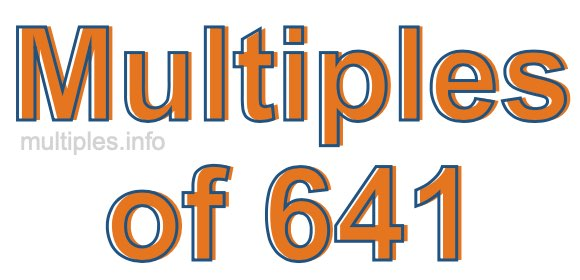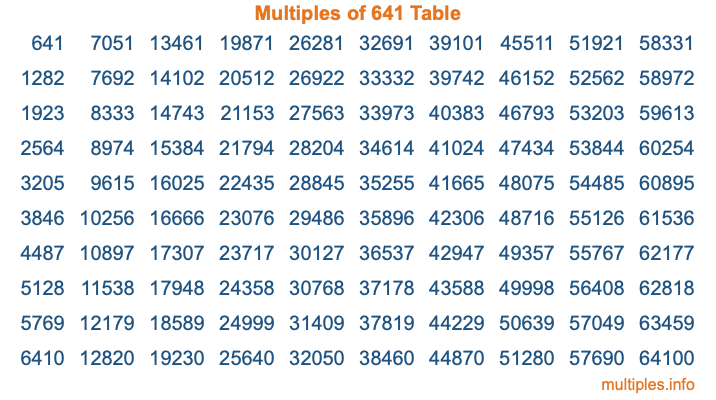Multiples of 641Welcome to the Multiples of 641 page. Here we will first teach you everything you will ever need to know about the multiples of 641, and then give you a study guide summary of everything we taught you to make sure you remember it all. Use this page to look up facts and learn information about the multiples of 641. This page will make you a multiples of six hundred forty-one expert!

Definition of Multiples of 641
Multiples of 641 are all the numbers that when divided by 641 equal an integer. Each of the multiples of 641 are called a multiple. A multiple of 641 is created by multiplying 641 by an integer.

Therefore, to create a list of multiples of 641, you start with 1 multiplied by 641, then 2 multiplied by 641, then 3 multiplied by 641, and so on for as long as you want. Thus, the list of the first five multiples of 641 is 641, 1282, 1923, 2564, and 3205. To see a larger list of multiples of 641, see the printable image of Multiples of 641 further down on this page. We also have a category where you can choose any nth multiple of 641.

Multiples of 641 Checker
The Multiples of 641 Checker below checks to see if any number of your choice is a multiple of 641. In other words, it checks to see if there is any number (integer) that when multiplied by 641 will equal your number. To do that, we divide your number by 641. If the the quotient is an integer, then your number is a multiple of 641.

Is  a multiple of 641?

Least Common Multiple of 641 and ...
A Least Common Multiple (LCM) is the lowest multiple that two or more numbers have in common. This is also called the smallest common multiple or lowest common multiple and is useful to know when you are adding our subtracting fractions. Enter one or more numbers below (641 is already entered) to find the LCM.

Check out our LCM Calculator if you need more details about the Least Common Multiple or if you need the LCM for different numbers for adding and subtraction fractions.

nth Multiple of 641
As we stated above, 641 is the first multiple of 641, 1282 is the second multiple of 641, 1923 is the third multiple of 641, and so on. Enter a number below to find the nth multiple of 641.

th multiple of 641

Multiples of 641 vs Factors of 641
641 is a multiple of 641 and a factor of 641, but that is where the similarities end. All postive multiples of 641 are 641 or greater than 641. All positive factors of 641 are 641 or less than 641.

Below is the beginning list of multiples of 641 and the factors of 641 so you can compare:

Multiples of 641: 641, 1282, 1923, 2564, 3205, etc.

Factors of 641: 1, 641

As you can see, the multiples of 641 are all the numbers that you can divide by 641 to get a whole number. The factors of 641, on the other hand, are all the whole numbers that you can multiply by another whole number to get 641.

It's also interesting to note that if a number (x) is a factor of 641, then 641 will also be a multiple of that number (x).

Multiples of 641 vs Divisors of 641
The divisors of 641 are all the integers that 641 can be divided by evenly. Below is a list of the divisors of 641.

Divisors of 641: 1, 641

The interesting thing to note here is that if you take any multiple of 641 and divide it by a divisor of 641, you will see that the quotient is an integer.

Multiples of 641 Table
Below is an image of the first 100 multiples of 641 in a table. The table is in chronological order, column by column. The first column has the first ten multiples of 641, the second column has the next ten multiples of 641, and so on.The Multiples of 641 Table is also referred to as the 641 Times Table or Times Table of 641. You are welcome to print out our table for your studies.

Negative Multiples of 641
Although not often discussed or needed in math, it is worth mentioning that you can make a list of negative multiples of 641 by multiplying 641 by -1, then by -2, then by -3, and so on, to get the following list of negative multiples of 641:

-641, -1282, -1923, -2564, -3205, etc.

Multiples of 641 Summary
Below is a summary of important Multiples of 641 facts that we have discussed on this page. To retain the knowledge on this page, we recommend that you read through the summary and explain to yourself or a study partner why they hold true.

There are an infinite number of multiples of 641.

A multiple of 641 divided by 641 will equal a whole number.

641 divided by a factor of 641 equals a divisor of 641.

The nth multiple of 641 is n times 641.

The largest factor of 641 is equal to the first positive multiple of 641.

641 is a multiple of every factor of 641.

641 is a multiple of 641.

A multiple of 641 divided by a divisor of 641 equals an integer.

641 divided by a divisor of 641 equals a factor of 641.

Any integer times 641 will equal a multiple of 641.

Multiples of a Number
Here you can get the multiples of another number, all with the same attention to detail as we did for multiples of 641 on this page.

Multiples of
Multiples of 642
Did you find our page about multiples of six hundred forty-one educational? Do you want more knowledge? Check out the multiples of the next number on our list!

Copyright  |   Privacy Policy  |   Disclaimer  |   Contact